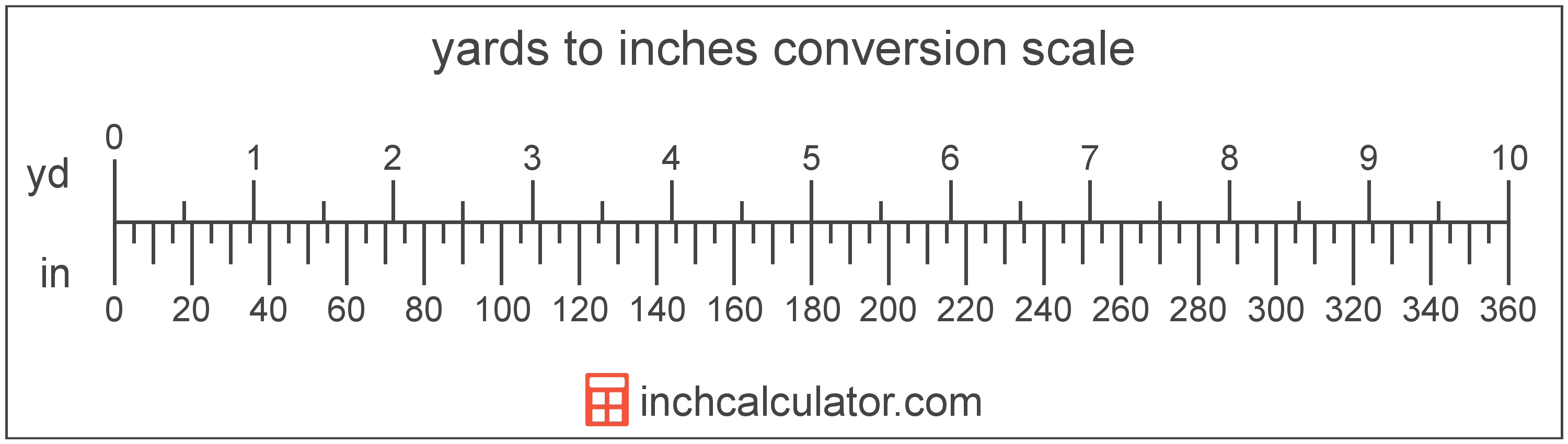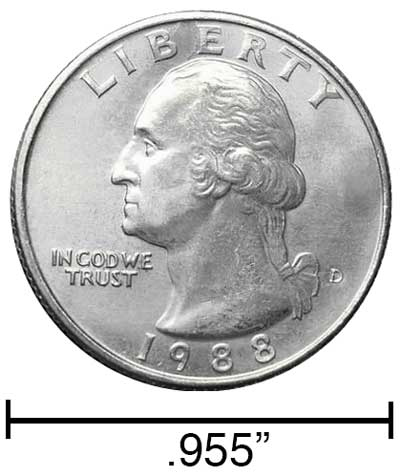# Yards to Inches Converter

Enter the length in yards below to get the value converted to inches.

yd
ft
Results in Inches:1 yd = 36 in

Do you want to convert inches to yards?

## How to Convert Yards to Inches

To convert a measurement in yards to a measurement in inches, multiply the length by the following conversion ratio: 36 inches/yard.

Since one yard is equal to 36 inches, you can use this simple formula to convert:

inches = yards × 36

The length in inches is equal to the length in yards multiplied by 36.

For example, here's how to convert 5 yards to inches using the formula above.
inches = (5 yd × 36) = 180"### How Many Inches Are in a Yard?

There are 36 inches in a yard, which is why we use this value in the formula above.

1 yd = 36"

Our inch fraction calculator can add yards and inches together, and it also automatically converts the results to US customary, imperial, and SI metric values.

## What Is a Yard?

The yard is a unit of length measurement equal to 3 feet or 36 inches. The international yard is legally defined to be equal to exactly 0.9144 meters.

The yard is a US customary and imperial unit of length. Yards can be abbreviated as yd; for example, 1 yard can be written as 1 yd.

## What Is an Inch?

An inch is a unit of length equal to 1/12 of a foot or 1/36 of a yard. Because the international yard is legally defined to be equal to exactly 0.9144 meters, one inch is equal to 2.54 centimeters.

The inch is a US customary and imperial unit of length. Inches can be abbreviated as in; for example, 1 inch can be written as 1 in.

Inches can also be denoted using the symbol, otherwise known as a double-prime. Often a double-quote (") is used instead of a double-prime for convenience. A double-prime is commonly used to express 1 in as 1″.The standard ruler is 12 inches long and is a common tool for measuring a length in inches. Another frequently used tool to perform measurements in inches is a tape measure, which commonly comes in lengths from 6' - 35'. Other types of devices used to make measurements in inches include scales, calipers, measuring wheels, micrometers, yardsticks, and even lasers.

We recommend using a ruler or tape measure for measuring length, which can be found at a local retailer or home center. Rulers are available in imperial, metric, or a combination of both values, so make sure you get the correct type for your needs.

Need a ruler? Try our free downloadable and printable rulers, which include both imperial and metric measurements.

## Yard to Inch Conversion Table

Table showing various yard measurements converted to inches.
Yards Inches
1 yd 36"
2 yd 72"
3 yd 108"
4 yd 144"
5 yd 180"
6 yd 216"
7 yd 252"
8 yd 288"
9 yd 324"
10 yd 360"
11 yd 396"
12 yd 432"
13 yd 468"
14 yd 504"
15 yd 540"
16 yd 576"
17 yd 612"
18 yd 648"
19 yd 684"
20 yd 720"
21 yd 756"
22 yd 792"
23 yd 828"
24 yd 864"
25 yd 900"
26 yd 936"
27 yd 972"
28 yd 1,008"
29 yd 1,044"
30 yd 1,080"
31 yd 1,116"
32 yd 1,152"
33 yd 1,188"
34 yd 1,224"
35 yd 1,260"
36 yd 1,296"
37 yd 1,332"
38 yd 1,368"
39 yd 1,404"
40 yd 1,440"

## References

1. National Bureau of Standards, Refinement of Values for the Yard and the Pound, https://www.ngs.noaa.gov/PUBS_LIB/FedRegister/FRdoc59-5442.pdf
2. National Institute of Standards and Technology, Checking the Net Contents of Packaged Goods, Handbook 133 - 2019 Edition, https://nvlpubs.nist.gov/nistpubs/hb/2019/NIST.HB.133-2019.pdf